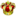• 0 Vote(s) - 0 Average
• 1
• 2
• 3
• 4
• 5
How Does MonsterMMORPG Catch - Capture Monster By Monster Box System Works
#1
1) Monsters can be captured only in wild Monster battles which you encounter while roaming on the maps

2) In order to capture a wild Monster during the battle you have to throw a Monster Box to the enemy wild Monster

3) In MonsterMMORPG in order to throw a Monster Box, you have to decrease enemy wild Monster HP to a certain percentage first

4) The percentages are like below

Regular Class: 100% or below of maximum HP

Superior Class: 90% or below of maximum HP

Emissary Class: 80% or below of maximum HP

Zenith Class: 75% or below of maximum HP

Starter Class: 75% or below of maximum HP

Legendary Class: 70% or below of maximum HP

Ancient Class: 60% or below of maximum HP

5) Once you have decreased enemy Monster's HP to the necessary percentage or below, you can throw any Monster Box you own

6) Then the following algorithm is run and whether you have captured the enemy Monster or not is decided

7) The lower the enemy wild Monster HP is, the more chance your capture try will be successful

8) Status effect

No negative status effect : 1x capture rate (variable_1)

sleep : 2x

freeze : 2x

paralyze : 1.5x

poison : 1.5x

burn : 1.5x

bleeding : 1.5x

The status effects are multiplied. For example if enemy Monster is under sleep and freeze effect, you will 4x more likely capture

9) Used Monster Box capture rate (variable_2)

For example regular Monster Box : 1x and Great Box : 1.5x

10) Enemy Monster's current HP and maximum HP (variable_3)

variable_3  = (MaximumHP * 3) - (Current HP * 2.5)

11) Catch rate of Monster written in MonsterDex page (variable_4)

For example Gryvolt has Catch Rate : 2 > https://www.monstermmorpg.com/Gryvolt-Monster-Dex-2137

Simoly has Catch Rate : 30 > https://www.monstermmorpg.com/Simoly-Monster-Dex-281

12) Enemy Monster Level (variable_5)

As your enemy Monster Level is lower, you are more likely to catch

It is calculated as (25 / Enemy Monster Level). So for example for level 5 wild Monster it is 5 and for level 100 wild Monster it is only 0.25

13) Variable_6 is calculated as following

Maximum HP of Monster * 4

14) Variable_7 is calculated as (Maximum HP / (Current HP * 4.0))

15) Variable_8 is calculated as following

Variable_8 = variable_3 * variable_4 * variable_2 * variable_1 * variable_5 / variable_6

16) If variable_8 is bigger than 300, you have successfully captured the enemy Monster

17) If variable_8 is lower than 300, it continues like below

18) variable_9 = a random integer between 1 and 240

variable_10 = a random integer between 1 and 240

variable_11 = a random integer between 1 and 240

variable_12 = a random integer between 1 and 240

19) if variable_8 is bigger than all of the variable_9 and variable_10 and variable_11 and variable_12 you have successfully captured the enemy wild Monster

20) There is a 1 exception for this

If you use Ultimate Box, you never fail

Forum Jump:

Users browsing this thread: 1 Guest(s)

Users browsed this thread:CeFurkan , GhostIsATroll , Monster King